## Use Commutators to Derive HO Energies

We have computed the commutatorsApply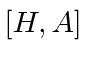to the energy eigenfunction.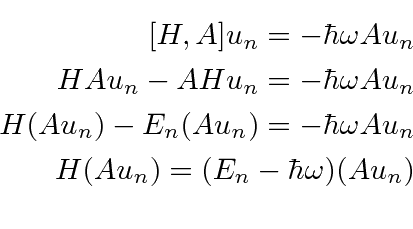This equation shows that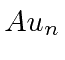is an eigenfunction of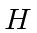with eigenvalue. Therefore,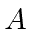lowers the energy by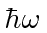.

Now, applyto the energy eigenfunction.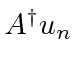is an eigenfunction ofwith eigenvalue.raises the energy by.

We cannot keep lowering the energy because the HO energy cannot go below zero.The only way to stop the lowering operator from taking the energy negative, is for the lowering to give zero for the wave function. Because this will be at the lowest energy, this must happen for the ground state. When we lower the ground state, we must get zero.Since the Hamiltonian containsin a convenient place, we can deduce the ground state energy.The ground state energy isand the states in general have energiessince we have shown raising and lowering in steps of. Only a state with energycan stop the lowering so the only energies allowed areIt is interesting to note that we have a number operator for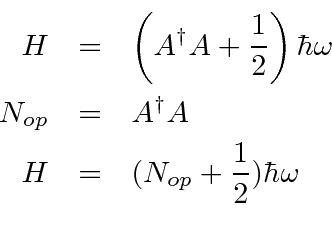Subsections
Jim Branson 2013-04-22